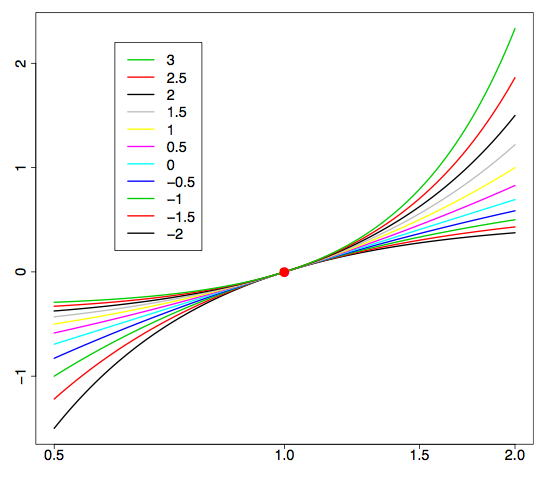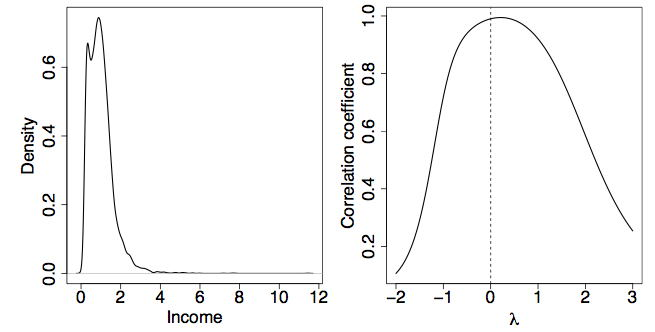# 16.4: Box-Cox Transformations

•• Contributed by David Lane
• Associate Professor (Psychology, Statistics, and Management) at Rice University

Learning Objectives

• To study the Box-Cox transformation

George Box and Sir David Cox collaborated on one paper (Box, $$1964$$). The story is that while Cox was visiting Box at Wisconsin, they decided they should write a paper together because of the similarity of their names (and that both are British). In fact, Professor Box is married to the daughter of Sir Ronald Fisher.

The Box-Cox transformation of the variable $$x$$ is also indexed by $$λ$$, and is defined as

$x' = \dfrac{x^\lambda-1}{\lambda} \label{eq1}$

At first glance, although the formula in Equation \ref{eq1} is a scaled version of the Tukey transformation $$x^\lambda$$, this transformation does not appear to be the same as the Tukey formula in Equation (2). However, a closer look shows that when $$λ < 0$$, both $$x_\lambda$$ and $$X_{\lambda }^{'}$$ change the sign of $$x^\lambda$$ to preserve the ordering. Of more interest is the fact that when $$λ = 0$$, then the Box-Cox variable is the indeterminate form $$0/0$$. Rewriting the Box-Cox formula as

$X_{\lambda }^{'}=\frac{e^{\lambda \log (x)}-1}{\lambda }\approx \frac{\left ( 1+\lambda \log (x) + \tfrac{1}{2}\lambda ^2\log (x)^2 + \cdots \right )-1}{\lambda }\rightarrow \log (x)$

as $$\lambda \rightarrow 0$$. This same result may also be obtained using l'Hôpital's rule from your calculus course. This gives a rigorous explanation for Tukey's suggestion that the log transformation (which is not an example of a polynomial transformation) may be inserted at the value $$λ = 0$$.Figure $$\PageIndex{1}$$: Examples of the Box-Cox transformation $$X_{\lambda }^{'}$$ versus $$x$$ for $$λ = −1, 0, 1$$. In the second row, $$X_{\lambda }^{'}$$ is plotted against $$log(x)$$. The red point is at $$(1, 0)$$.

Notice with this definition of $$X_{\lambda }^{'}$$ that $$x = 1$$ always maps to the point $$X_{\lambda }^{'} = 0$$ for all values of $$λ$$. To see how the transformation works, look at the examples in Figure $$\PageIndex{1}$$. In the top row, the choice $$λ = 1$$ simply shifts $$x$$ to the value $$x−1$$, which is a straight line. In the bottom row (on a semi-logarithmic scale), the choice $$λ = 0$$ corresponds to a logarithmic transformation, which is now a straight line. We superimpose a larger collection of transformations on a semi-logarithmic scale in Figure $$\PageIndex{2}$$.Figure $$\PageIndex{2}$$: Examples of the Box-Cox transformation $$X_{\lambda }^{'}$$ versus $$log(x)$$ for $$−2 < λ < 3$$. The bottom curve corresponds to $$λ = −2$$ and the upper to $$λ = 3$$.

## Transformation to Normality

Another important use of variable transformation is to eliminate skewness and other distributional features that complicate analysis. Often the goal is to find a simple transformation that leads to normality. In the article on $$q-q$$ plots, we discuss how to assess the normality of a set of data,

$x_1,x_2, \ldots ,x_n.$

Data that are normal lead to a straight line on the q-q plot. Since the correlation coefficient is maximized when a scatter diagram is linear, we can use the same approach above to find the most normal transformation.

Specifically, we form the $$n$$ pairs

$\left ( \Phi ^{-1} \left ( \frac{i-0.5}{n} \right ), x_{(i)} \right ),\; for\; i=1,2,\cdots ,n$

where $$\Phi ^{-1}$$ is the inverse CDF of the normal density and $$x_{(i)}$$ denotes the $$i^{th}$$ sorted value of the data set. As an example, consider a large sample of British household incomes taken in $$1973$$, normalized to have mean equal to one ($$n = 7125$$). Such data are often strongly skewed, as is clear from Figure $$\PageIndex{3}$$. The data were sorted and paired with the $$7125$$ normal quantiles. The value of $$λ$$ that gave the greatest correlation ($$r = 0.9944$$) was $$λ = 0.21$$.Figure $$\PageIndex{3}$$: (L) Density plot of the $$1973$$ British income data. (R) The best value of $$λ$$ is $$0.21$$.

The kernel density plot of the optimally transformed data is shown in the left frame of Figure $$\PageIndex{4}$$. While this figure is much less skewed than in Figure $$\PageIndex{3}$$, there is clearly an extra "component" in the distribution that might reflect the poor. Economists often analyze the logarithm of income corresponding to $$λ = 0$$; see Figure $$\PageIndex{4}$$. The correlation is only $$r = 0.9901$$ in this case, but for convenience, the log-transform probably will be preferred.Figure $$\PageIndex{4}$$: (L) Density plot of the $$1973$$ British income data transformed with $$λ = 0.21$$. (R) The log-transform with $$λ = 0$$.

## Other Applications

Regression analysis is another application where variable transformation is frequently applied. For the model

$y =\beta_o + \beta_1 x_1 + \beta_2 x_2 + \ldots \beta_p x_p + \epsilon$

and fitted model

$\widehat{y}=b_0 + b_1x_1 + b_2x_2 + \cdots + b_px_p$

each of the predictor variables $$x_j$$ can be transformed. The usual criterion is the variance of the residuals, given by

$\frac{1}{n} \sum_{i=1}^{n} (\widehat{y}_i-y_i)^2$

Occasionally, the response variable y may be transformed. In this case, care must be taken because the variance of the residuals is not comparable as $$λ$$ varies. Let $$\bar{g}_y$$ represent the geometric mean of the response variables.

$\bar{g}_y = \left ( \prod_{i-1}^{n} y_i \right )^{1/n}$

Then the transformed response is defined as

$y_{\lambda }^{'} = \frac{y^\lambda -1}{\lambda \cdot \bar{g}_{y}^{\lambda -1}}$

When $$λ = 0$$ (the logarithmic case),

$y_{0}^{'} = \bar{g}_y \cdot \log (y)$

For more examples and discussions, see Kutner, Nachtsheim, Neter, and Li (2004).

## References

1. Box, G. E. P. and Cox, D. R. (1964). An analysis of transformations, Journal of the Royal Statistical Society, Series B, 26, 211-252.
2. Kutner, M., Nachtsheim, C., Neter, J., and Li, W. (2004). Applied Linear Statistical Models, McGraw-Hill/Irwin, Homewood, IL.